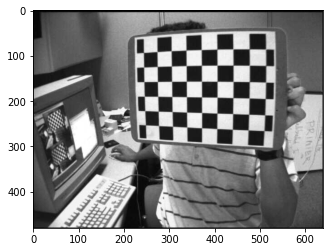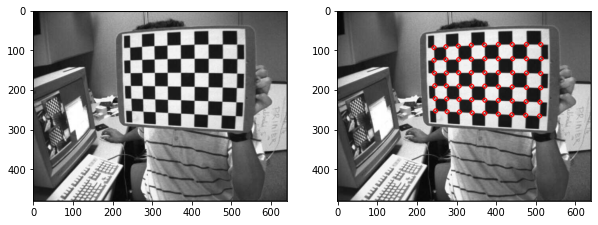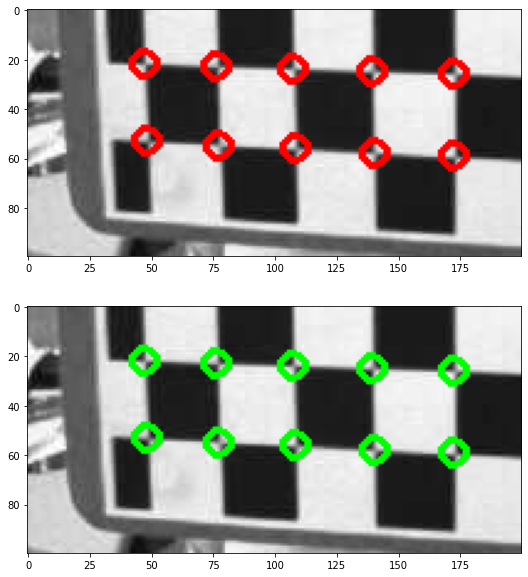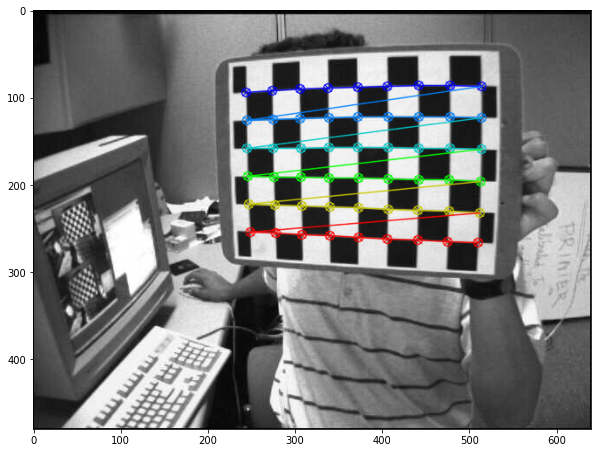# Camera Calibration in OpenCV¶

Faisal Qureshi
Professor
Faculty of Science
Ontario Tech University
http://vclab.science.ontariotechu.ca

## Outline¶

• OpenCV checkerboard based camera calibration
• Image undistortion

Chessboards are frequently used as test images for camera calibration in computer vision.

The task here is to use utilities bundled with OpenCV to calibrate a camera from a set of chessboard images.

## Camera calibration using a checkerboard pattern¶

In :
import numpy as np
import cv2 as cv
import matplotlib.pyplot as plt


The directory data contains a set of images left01.jpg, left02.jpg, ... left14.jpg that can be used for camera calibration. Use a glob pattern to create a list images of all the image filepaths (i.e., a list of strings).

In :
# Your solution here

In :
# %load solutions/camera-calibration/solution_01.py
# creates a list of strings of filepaths to image files
import glob
images = sorted(glob.glob('data/left??.jpg'))
print(images)


#### Exercise: examining the first image¶

Extract the first image filename from the list images and convert it to a grayscale image.

• Use cv.imread to load the image.
• Use cv.cvtColor to convert the image from RGB to grayscale.
• Use the identifier img for the original image & gray for the grayscale version
• Use plt.imshow to visualize the image gray.
In :
# Your solution here

In :
# %load solutions/camera-calibration/solution_02.py
# loading an image from the previously constructed list of filepaths images.
img = cv.imread(images) # Extract the first image as img
print(img.shape)
gray = cv.cvtColor(img, cv.COLOR_BGR2GRAY) # Convert to a gray scale image
print(img.shape, gray.shape)
plt.imshow(gray, cmap='gray'); # Visualize gray

(480, 640, 3)
(480, 640, 3) (480, 640)#### Exercise: examining the first image¶

• Use the findChessboardCorners function built-in to OpenCV to extract the corners from the image gray.
• Assume a pattern of size (9,6) (corresponding to the interior corners to locate in the chessboard).
• Assign the output array of corner coordinates to the identifier corners.
• Use NumPy's squeeze function to eliminate singleton dimensions from the array corners.

We'll see later how corner detection is actually done.

In :
# Your solution here

In :
# %load solutions/camera-calibration/solution_03.py
# Extract the corners and the return value
retval, corners = cv.findChessboardCorners(image=gray, patternSize=(9,6))
print(corners.shape)
corners = np.squeeze(corners) # Get rid of extraneous singleton dimension
print(corners.shape)
print(corners[:5])  #Examine the first few rows of corners

(54, 1, 2)
(54, 2)
[[244.45415   94.33141 ]
[274.62177   92.24126 ]
[305.49387   90.402885]
[338.36407   88.836266]
[371.59216   87.98364 ]]


With the image img and the array corners, we can now produce a figure showing the original image and the image with circles overlaid on the corner coordinates.

In :
img2 = np.copy(img)  # Make a copy of original img as img2

# Add circles to img2 at each corner identified
for corner in corners:
coord = (corner, corner)
cv.circle(img=img2, center=coord, radius=5, color=(255, 0, 0), thickness=2)

# Produce a figure with the original image img in one subplot and modified image img2 (with the corners added in).
plt.figure(figsize=(10,10))
plt.subplot(121)
plt.imshow(img)
plt.subplot(122)
plt.imshow(img2);The cornerSubPix function from OpenCV can be used to refine the corners extracted to sub-pixel accuracy. This is based on an iterative technique; as such, one of the inputs criteria uses a tuple to bundle a convergence tolerance and a maximum number of iterations.

In :
criteria = (cv.TERM_CRITERIA_EPS + cv.TERM_CRITERIA_MAX_ITER, 30, 0.001) # Set termination criteria as a tuple.
corners_orig = corners.copy()  # Preserve the original corners for comparison after
corners = cv.cornerSubPix(gray, corners, (11,11), (-1,-1), criteria=criteria) # extract refined corner coordinates.

In :
# Examine how much the corners have shifted (in pixels)
shift = corners - corners_orig
print(shift[:4,:])
print(np.linalg.norm(shift.reshape(-1,1), np.inf))

[[-0.0488739  -0.19455719]
[-0.22705078 -0.03068542]
[ 0.0071106  -0.08568573]
[-0.05487061 -0.04328918]]
0.38919067


Now, generate a figure to compare the original corners to the corrected corners.

In :
img3 = np.copy(img)

for corner in corners:
coord = (corner, corner)
cv.circle(img=img3, center=coord, radius=5, color=(0, 255, 0), thickness=2)

plt.figure(figsize=(10,10))
plt.subplot(211)
plt.imshow(img2[200:300,200:400,:])
plt.subplot(212)
plt.imshow(img3[200:300,200:400,:]);The function drawChessboardCorners generates a new image with circles at the corners detected. The corners are displayed either as red circles if the board was not found, or as colored corners connected with lines if the board was found (as determined by the output argument retval from findChessboardCorners).

In :
img4 = cv.drawChessboardCorners(img, (9, 6), corners, retval)
plt.figure(figsize=(10,10))
plt.imshow(img4);Finally, we're going to repeat this process with all the chessboard images to remove distortion effects. First, assume a 3d world coordinate system aligned with the chessboard.

In :
obj_grid = np.zeros((9*6,3), np.float32)
obj_grid[:,:2] = np.mgrid[0:9,0:6].T.reshape(-1,2)
print(obj_grid)

[[0. 0. 0.]
[1. 0. 0.]
[2. 0. 0.]
[3. 0. 0.]
[4. 0. 0.]
[5. 0. 0.]
[6. 0. 0.]
[7. 0. 0.]
[8. 0. 0.]
[0. 1. 0.]
[1. 1. 0.]
[2. 1. 0.]
[3. 1. 0.]
[4. 1. 0.]
[5. 1. 0.]
[6. 1. 0.]
[7. 1. 0.]
[8. 1. 0.]
[0. 2. 0.]
[1. 2. 0.]
[2. 2. 0.]
[3. 2. 0.]
[4. 2. 0.]
[5. 2. 0.]
[6. 2. 0.]
[7. 2. 0.]
[8. 2. 0.]
[0. 3. 0.]
[1. 3. 0.]
[2. 3. 0.]
[3. 3. 0.]
[4. 3. 0.]
[5. 3. 0.]
[6. 3. 0.]
[7. 3. 0.]
[8. 3. 0.]
[0. 4. 0.]
[1. 4. 0.]
[2. 4. 0.]
[3. 4. 0.]
[4. 4. 0.]
[5. 4. 0.]
[6. 4. 0.]
[7. 4. 0.]
[8. 4. 0.]
[0. 5. 0.]
[1. 5. 0.]
[2. 5. 0.]
[3. 5. 0.]
[4. 5. 0.]
[5. 5. 0.]
[6. 5. 0.]
[7. 5. 0.]
[8. 5. 0.]]

In :
# Initialize enpty list to accumulate coordinates
obj_points = [] # 3d world coordinates
img_points = [] # 2d image coordinates

In :
criteria = (cv.TERM_CRITERIA_EPS + cv.TERM_CRITERIA_MAX_ITER, 30, 0.001)

for fname in images:
gray = cv.cvtColor(img, cv.COLOR_BGR2GRAY)

retval, corners = cv.findChessboardCorners(gray, (9,6))
if retval:
obj_points.append(obj_grid)
corners2 = cv.cornerSubPix(gray, corners, (11,11), (-1,-1), criteria)
img_points.append(corners2)

Loading data/left01.jpg


The accumulated lists of object coordinates and image coordinates can be combined to determine an optimal set of camera calibration parameters. The relevant OpenCV utility here is calibrateCamera.

In :
retval, mtx, dist, rvecs, tvecs = cv.calibrateCamera(obj_points, img_points, gray.shape[::-1], None, None)

In :
print(retval) # Objective function value
print(mtx)    # Camera matrix
print(dist)   # Distortion coefficients

0.4086955848818598
[[536.07343021   0.         342.37038771]
[  0.         536.01634477 235.5368565 ]
[  0.           0.           1.        ]]
[[-0.26509006 -0.0467439   0.00183301 -0.00031471  0.25231586]]


The function getOptimalNewCameraMatrix can use the optimized matrix and distortion coefficients to construct a new camera matrix appropriate for a given image. This can be used to remove distortion effects with undistort.

In :
img = cv.imread('data/left12.jpg')
h,w = img.shape[:2]
newcameramtx, roi = cv.getOptimalNewCameraMatrix(mtx, dist, (w,h), 1, (w,h))

In :
# undistort
dst = cv.undistort(img, mtx, dist, None, newcameramtx)

# crop the image
x,y,w,h = roi
dst = dst[y:y+h, x:x+w]

In :
plt.figure(figsize=(10,10))
plt.subplot(121)
plt.imshow(img)
plt.title('Original')
plt.subplot(122)
plt.imshow(dst)
plt.title('Corrected');